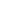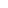Blender Python Script:  Modifying a Square Mesh  (Part 4): Automating Face Creation.

Version Française
 Main Index                                       Python Index Previous Python (3): Automating Vertices Creation (3) Python (5): Potatoid Next

Creating the faces is not much more difficult than that of the vertices. However, it is necessary to observe which vertices will have to be assembled and in which order. We start, once again, by using the same variables n , i and j . Though, this time, i and j represent the row and column position of the faces, respectively. As before, we proceed, one line at a time.

For i = 0 and j = 0, the vertices are:

verts[ 0 ],
verts[ 1 ],
verts[ 4 ],
verts[ 3 ],
which can be transformed into:
verts[ i*n+j ],
verts[ i*n+j+1 ],
verts[ (i+1)*n+j+1 ],
verts[ (i+1)*n+j ]
One quickly realizes that all the faces can be filled in, while varying i and j between 0.0 and n - 1. The remainder is only a matter of filling in the blanks. Well, almost; it is also necessary to transform the value n into an integer ; since the indices of a list can only be integers. The following method is perhaps not very elegant, but it does illustrate some techniques which we have already discussed :
n0=len(range(0, n))
Python offers a more succinct conversion function, to convert the type as follows:
n0=int(n)
i=0, j=0
 012345678
i=0, j=1
 012345678
i=1, j=0
 012345678
i=1, j=1
 012345678

Python script source:

 # [ snip... ]  # we resume at the exact location in the source  # where we left off from in the previous part. # Translate the value of n from a floating point  # (decimal) value to an integer (whole number).  # n0=len(range(0, n)) n0=int(n) for i in range(0, n-1):        # The first subroutine begins here,        # with four spaces of indentation        for j in range(0, n-1):            # The second loop begins here,            # with eight spaces of indentation            f=NMesh.Face()            f.v.append(me.verts[i*n0+j ])            f.v.append(me.verts[i*n0+j+1 ])            f.v.append(me.verts[(i+1)*n0+j+1 ])            f.v.append(me.verts[(i+1)*n0+j ])           # After specifying the vertice co-ordinates for the           # face, it is added to the faces list of " me ".           me.faces.append(f)           # End of the internal loop        # End of the external loop  NMesh.PutRaw(me, " plane", 1)  Blender.Redraw() # To test the Python file

 Previous Python (3): automating vertices creation Python (5): Potatoid Next Top of page

 NOTE:   To prevent  a face from being crossed by an edge segment, the succession of vertices must precisely follow the perimeter of each individual face.